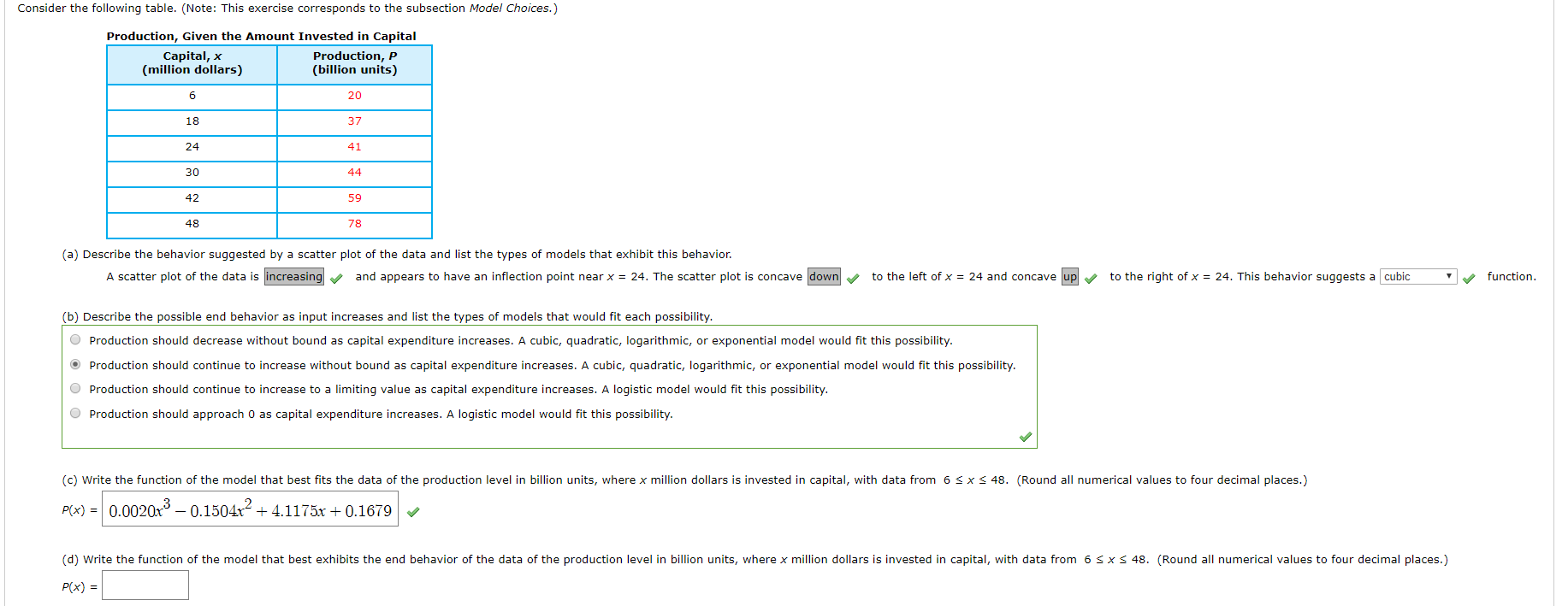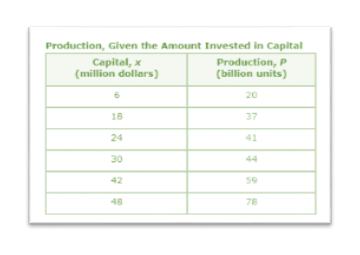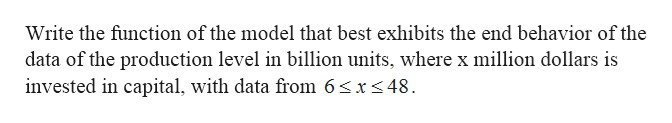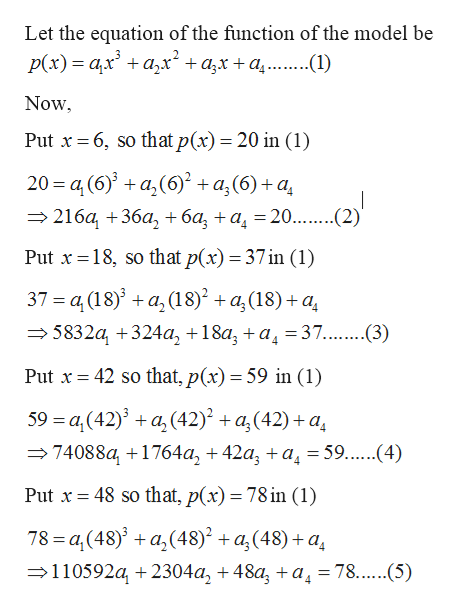# Consider the following table. (Note: This exercise corresponds to the subsection Model Choices.)Production, Given the Amount Invested in CapitalCapital, x(million dollars)Production, P(billion units)62018372441304442594878(a) Describe the behavior suggested by a scatter plot of the data and list the types of models that exhibit this behavior.A scatter plot of the data is increasingto the left of x 24 and concave upand appears to have an inflection point near x = 24. The scatter plot is concave downto the right of x = 24. This behavior suggests a cubicfunction(b) Describe the possible end behavior as input increases and list the types of models that would fit each possibility.Production should decrease without bound as capital expenditure increases. A cubic, quadratic, logarithmic, or exponential model would fit this possibilityProduction should continue to increase without bound as capital expenditure increases. A cubic, quadratic, logarithmic, or exponential model would fit this possibility.would fit this possibility.Production should continue to increase to a limiting value as capital expenditure increases. A logisticProduction should approach 0 as capital expenditure increases. A logistic model would fit this possibility.(c) Write the function of the model that best fits the data of the production level in billion units, where x million dollars is invested in capital, with data from 6 S x48. (Round all numerical values to four decimal places.)P(x) 0.0020x3-0.1504x24.1175x0.1679(d) Write the function of the model that best exhibits the end behavior of the data of the production level in billion units, where x million dollars is invested in capital, with data from 6 s x48. (Round all numerical values to four decimal places.)P(x)

Question

i am having trouble with d)help_outlineImage TranscriptioncloseConsider the following table. (Note: This exercise corresponds to the subsection Model Choices.) Production, Given the Amount Invested in Capital Capital, x (million dollars) Production, P (billion units) 6 20 18 37 24 41 30 44 42 59 48 78 (a) Describe the behavior suggested by a scatter plot of the data and list the types of models that exhibit this behavior. A scatter plot of the data is increasing to the left of x 24 and concave up and appears to have an inflection point near x = 24. The scatter plot is concave down to the right of x = 24. This behavior suggests a cubic function (b) Describe the possible end behavior as input increases and list the types of models that would fit each possibility. Production should decrease without bound as capital expenditure increases. A cubic, quadratic, logarithmic, or exponential model would fit this possibility Production should continue to increase without bound as capital expenditure increases. A cubic, quadratic, logarithmic, or exponential model would fit this possibility. would fit this possibility. Production should continue to increase to a limiting value as capital expenditure increases. A logistic Production should approach 0 as capital expenditure increases. A logistic model would fit this possibility. (c) Write the function of the model that best fits the data of the production level in billion units, where x million dollars is invested in capital, with data from 6 S x 48. (Round all numerical values to four decimal places.) P(x) 0.0020x3-0.1504x2 4.1175x0.1679 (d) Write the function of the model that best exhibits the end behavior of the data of the production level in billion units, where x million dollars is invested in capital, with data from 6 s x 48. (Round all numerical values to four decimal places.) P(x) fullscreen
check_circleExpert Solution
Step 1

Given: -help_outlineImage TranscriptioncloseProduction, Given the Amount Invested in Capital Capital, x (million dollers) Production, P (billion units) 20 18 37 24 41 30 44 42 59 48 78 fullscreen
Step 2

To find: -help_outlineImage TranscriptioncloseWrite the function of the model that best exhibits the end behavior of the data of the production level in billion units, where x million dollars is invested in capital, with data from 6sx<48 fullscreen
Step 3

Calculation...help_outlineImage TranscriptioncloseLet the equation of the function of the model be Р(x) %3 а,х' + а,х* + а,х +а,.. (1) Now Put x6, so that p(x) = 20 in (1) 20 a, (6)a,(6) a,(6)+a — 216а, +36а, + ба, +а, 20...(2) Put x18, so that p(x) = 37in (1) 37 = a, (18)a, (18) a(18)+a 5832a, 324a, +18a, +a =37...(3) Put 42 so that, p(x) = 59 in (1) 59 - а, (42)' + а, (42)* + а,(42) + а, 74088a, 1764a, +42a, +a = 59...(4) Put x48 so that, p(x) = 78 in (1) 78 a,(48) a(48)^ + a(48)+a 110592a 2304a, 48a, a = 78. ....5) fullscreen

### Want to see the full answer?

See Solution

#### Want to see this answer and more?

Solutions are written by subject experts who are available 24/7. Questions are typically answered within 1 hour*

See Solution
*Response times may vary by subject and question
Tagged in

### Functions